2019-06-30 17:06:07 weixin_38358881 阅读数 1074
• ###### 云从科技：详解CNN-pFSMN模型以及在语音识别中的应用

近日，云从科技在语音识别技术上取得重大突破，在全球最大的开源语音识别数据集Librispeech上刷新了世界纪录，错词率（Worderrorrate，WER）降到了2.97%，将Librispeech的WER指标提升了25%，大幅刷新原先记录。本视频课程讲解了：大规模词汇连续语音识别，LVCSR前沿与最新进展，和CNN-pFSMN声学模型。

1122 人正在学习 去看看 CSDN讲师

# 语音识别

LibriSpeech 数据集

## WER评价指标

WER means " word error rate"WER的取值范围是最小是0，最大可以大于1。此外，类似的评价指标还有SER，CER，仅仅是粗细粒度不同，计算方式相同。

2019-12-01 20:03:24 weixin_42697449 阅读数 125
• ###### 云从科技：详解CNN-pFSMN模型以及在语音识别中的应用

近日，云从科技在语音识别技术上取得重大突破，在全球最大的开源语音识别数据集Librispeech上刷新了世界纪录，错词率（Worderrorrate，WER）降到了2.97%，将Librispeech的WER指标提升了25%，大幅刷新原先记录。本视频课程讲解了：大规模词汇连续语音识别，LVCSR前沿与最新进展，和CNN-pFSMN声学模型。

1122 人正在学习 去看看 CSDN讲师

### 软件测试之语音识别(ASR)测试

**写在前面：测试不是一种目的，而是一种保证软件质量的手段**

在测试语音识别的过程中，衡量识别内容的准确性，一般通过如下指标进行评估

•     WER(Word Error Rate,词错率)

定义：为了使识别出来的词序列和标准的词序列之间保持一致，需要进行替换、删除或者插入某些词，这些插入、替换或删除的词的总个数，除以标准的词序列中词的总个数的百分比，即为WER

WER=(S+D+I)/N

S:substitution,替换

D:Deletion,删除

I:Insertion,插入

N:标准词序列中词的总个数

(S+D+I)=识别出来的词序列与标准的词序列之间的编辑距离

注意：因为有插入词(Insertion)，所以可能会出现WER>1的情况，不过，WER>1时，表明识别出来的词序列和标准的词序列相差很大，识别效果特别差

•   SER(Sentence Error Rate,句错率)

定义：句子中如果有一个词识别错误，那么这个句子被认为识别错误，句子识别错误的个数，除以总的句子个数即为SER

SER=SE/N

SE:识别出来的序列中，识别错误的句子个数（即WER!=0的句子个数）

N:标准序列中总句子个数

(1)、语音识别的测试点主要包括如下几点：

i.输入测试语音

（中间过程：语音识别模块接收到测试语音，执行语音转文字操作，并将识别文字输出）

ii.验证识别文字内容的准确性

iii.语音识别耗时

(2)、 测试语音的输入，主要包括以下几个方面：

①语音输入离拾音设备的距离：近距离、远距离

②拾音设备：移动端(安卓、IOS)原生麦克风、PC端原生麦克风、日常用耳机麦克风、专业设备麦克风(罗技等)

③语音输入与拾音设备的相对位置：正对拾音口，背对拾音口

④语音输入的环境：办公环境、会议室环境

⑤语音输入时的语速：正常语速、语速较快、语速较慢、时快时慢、断断续续

⑥语音输入时的音量：正常音量、音量较大、音量较小，时大时小

⑦语音输入时的音色：男声、女声

⑧语音输入的音频类型：自然人声、录音、广播、变声等

(3)、测试识别内容，主要包括以下几个方面：

①支持识别的语种：普通话、英文、方言等

②支持的应用场景：日常对话、尽职调查、娱乐访谈等

③支持的对话模式：单人对话，多人对话，同一时间只有一个人说话，同一时间多人一起说话

③覆盖的词库内容：根据应用场景，是否包含了应用场景(比如金融、娱乐领域)对应的词库训练

(4)、验证识别内容的准确性

统计识别文字的WER、SER指标

(5)、关注语音识别耗时

①短语音的识别耗时

②长语音的识别耗时

①计算识别的词序列与标准的词序列之间的编辑距离

②计算WER

③计算SER

2019-07-22 16:04:42 NIeson2012 阅读数 2350
• ###### 云从科技：详解CNN-pFSMN模型以及在语音识别中的应用

近日，云从科技在语音识别技术上取得重大突破，在全球最大的开源语音识别数据集Librispeech上刷新了世界纪录，错词率（Worderrorrate，WER）降到了2.97%，将Librispeech的WER指标提升了25%，大幅刷新原先记录。本视频课程讲解了：大规模词汇连续语音识别，LVCSR前沿与最新进展，和CNN-pFSMN声学模型。

1122 人正在学习 去看看 CSDN讲师

1.背景

2.孤立词识别

2.1 特征提取

2.2 动态弯算法

2.3 GMM(Gaussian mixture model)

2.4 HMM(Hidden markov model)

2.5 EM训练算法

2.6 语音识别基本方程

3.连续语音识别

3.1 语言模型

3.2 大词汇量

3.3 语音识别系统结构

3.4 评价指标：WER

4. 潘多拉魔盒

4.1 上下文有关模型

4.2 区分式训练

4.3 说话人适应

4.4 二次打分

5.结语

# 1.背景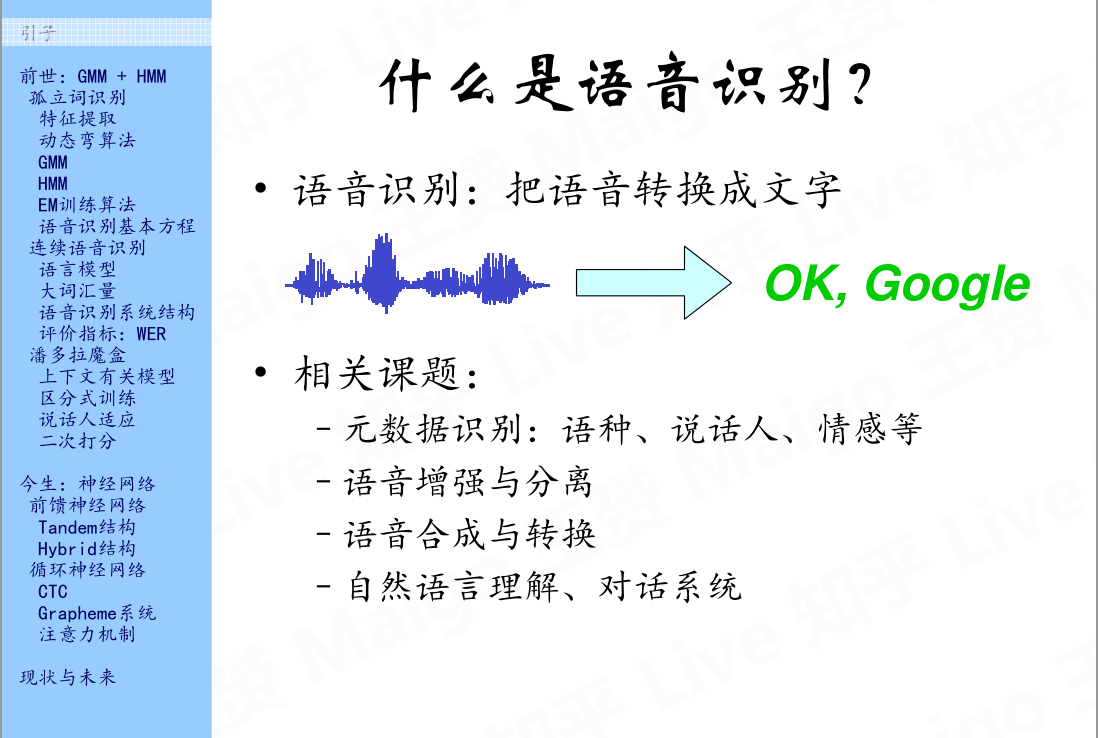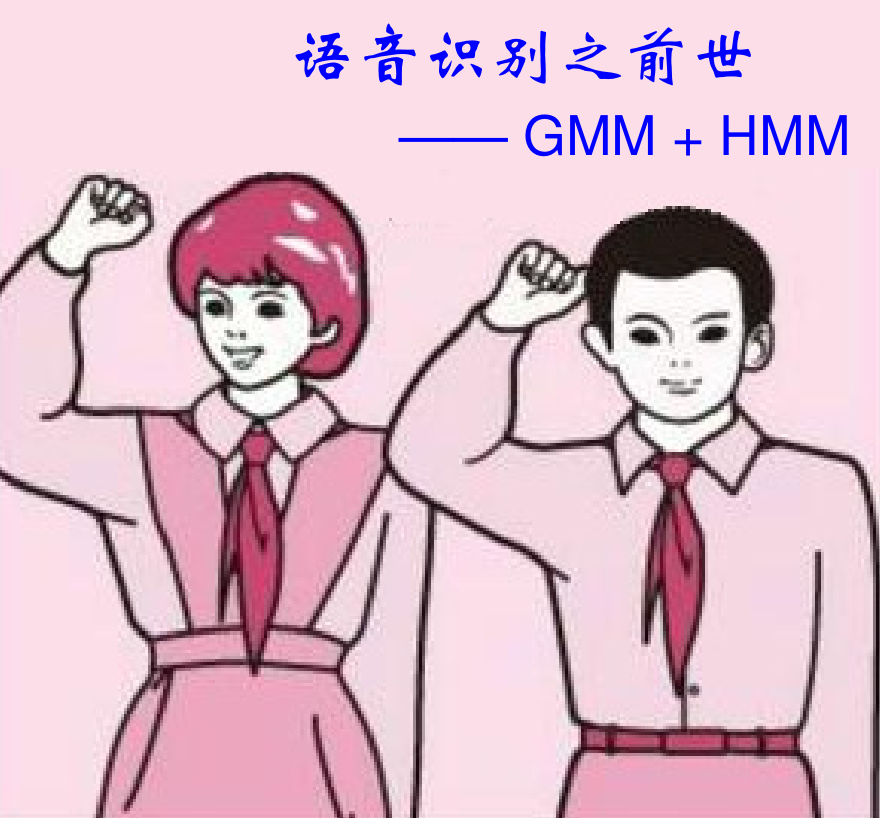# 2.孤立词识别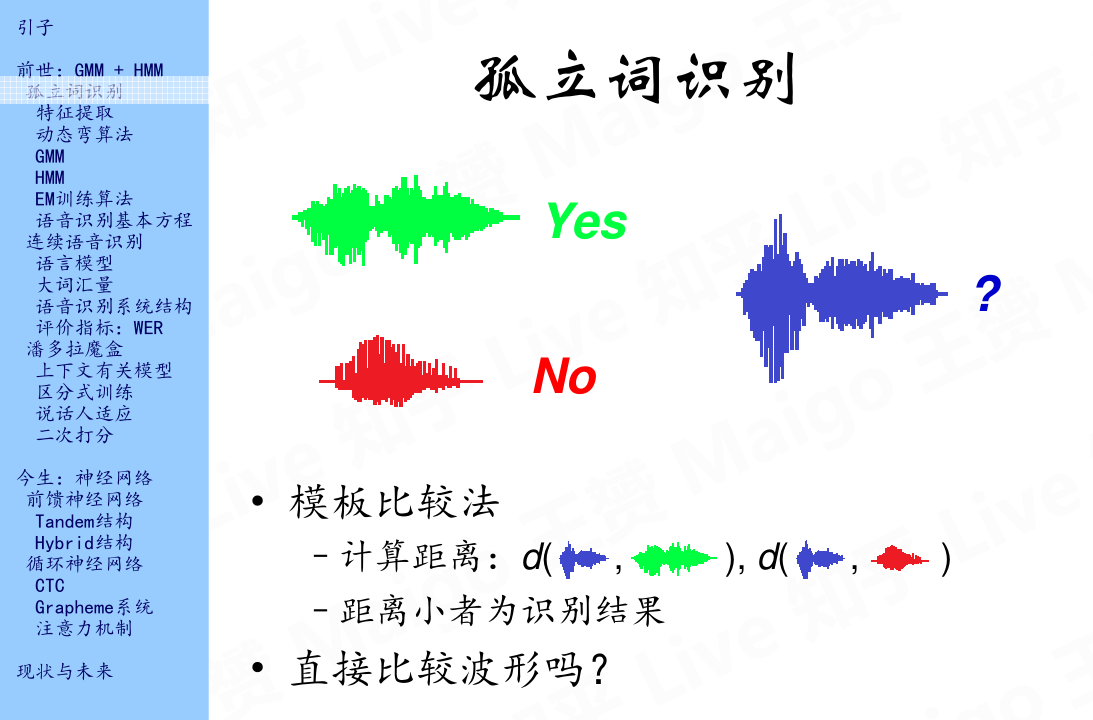## 2.1 特征提取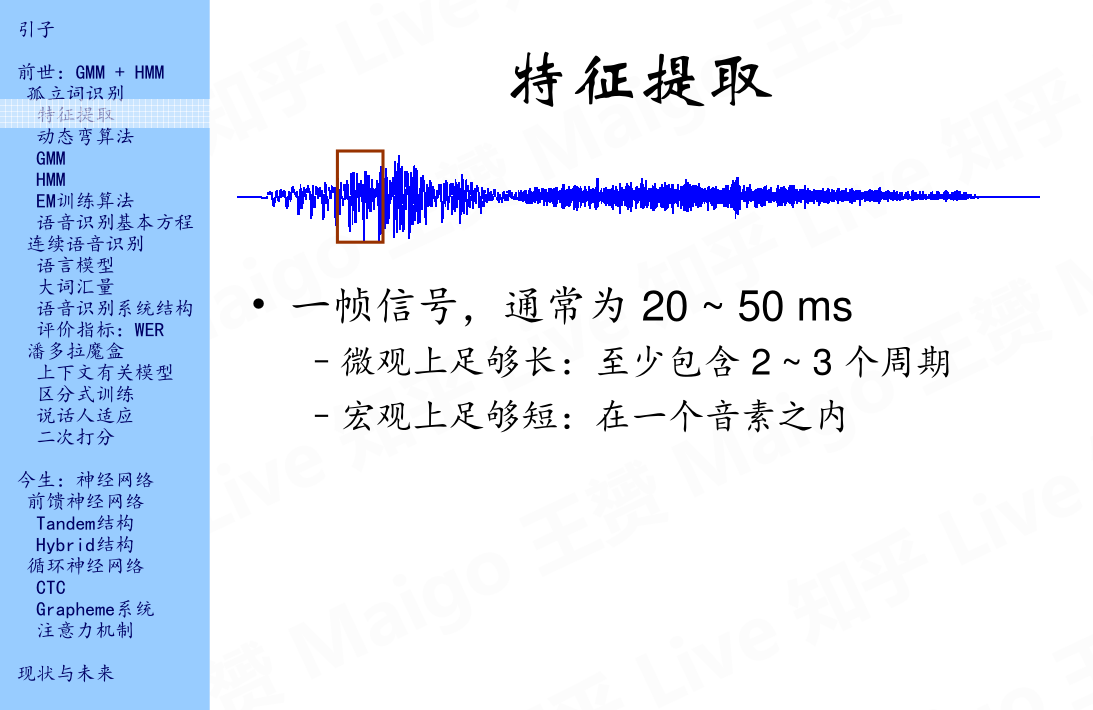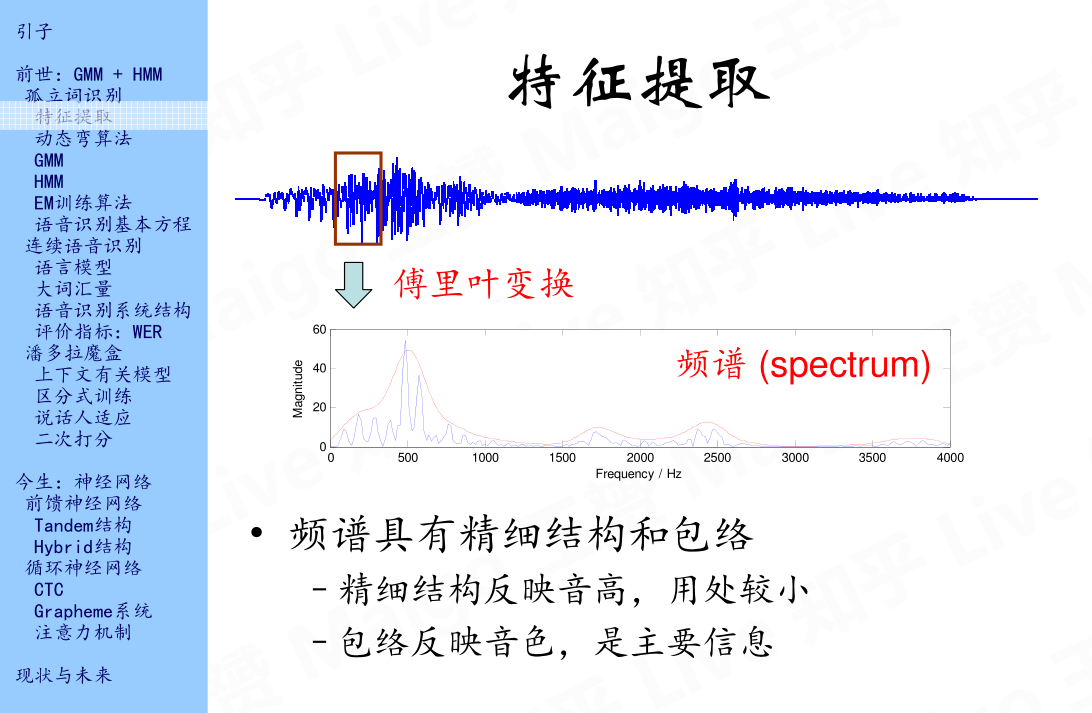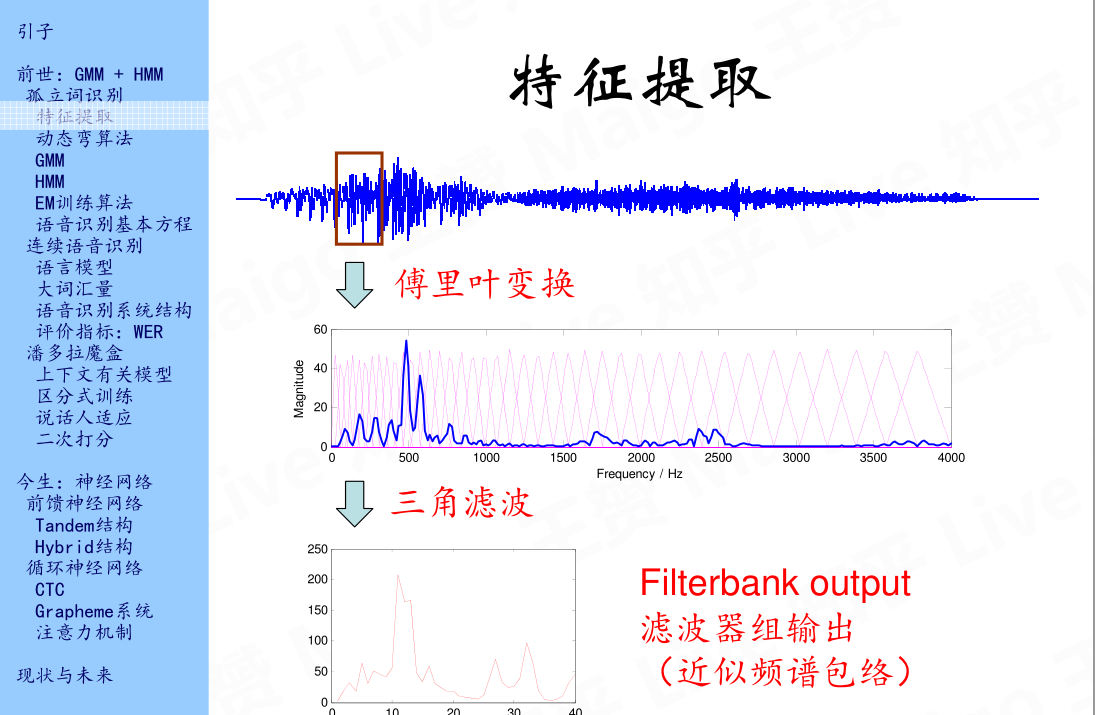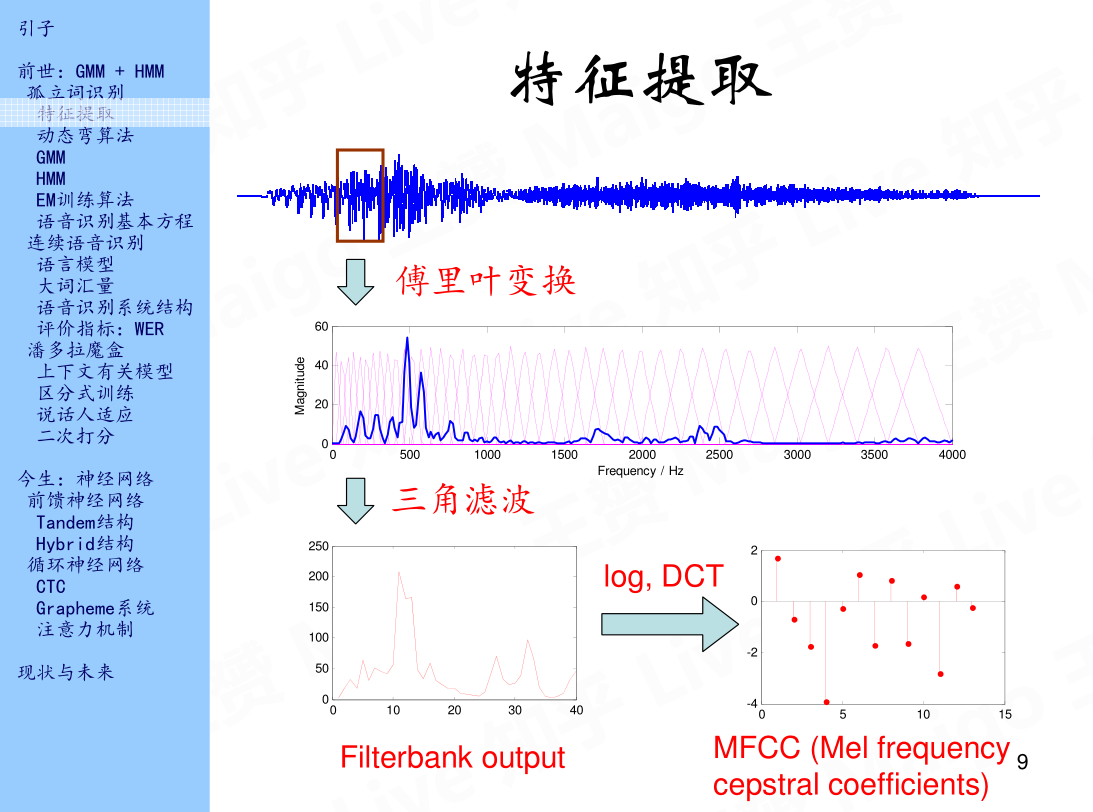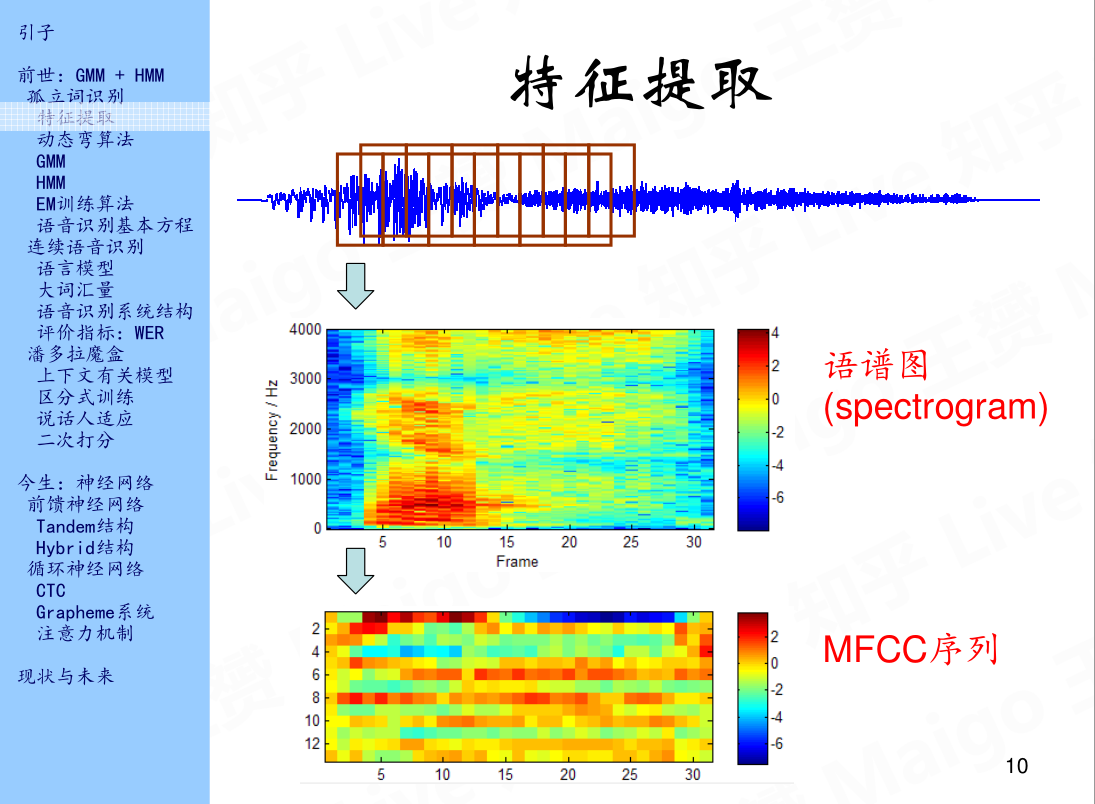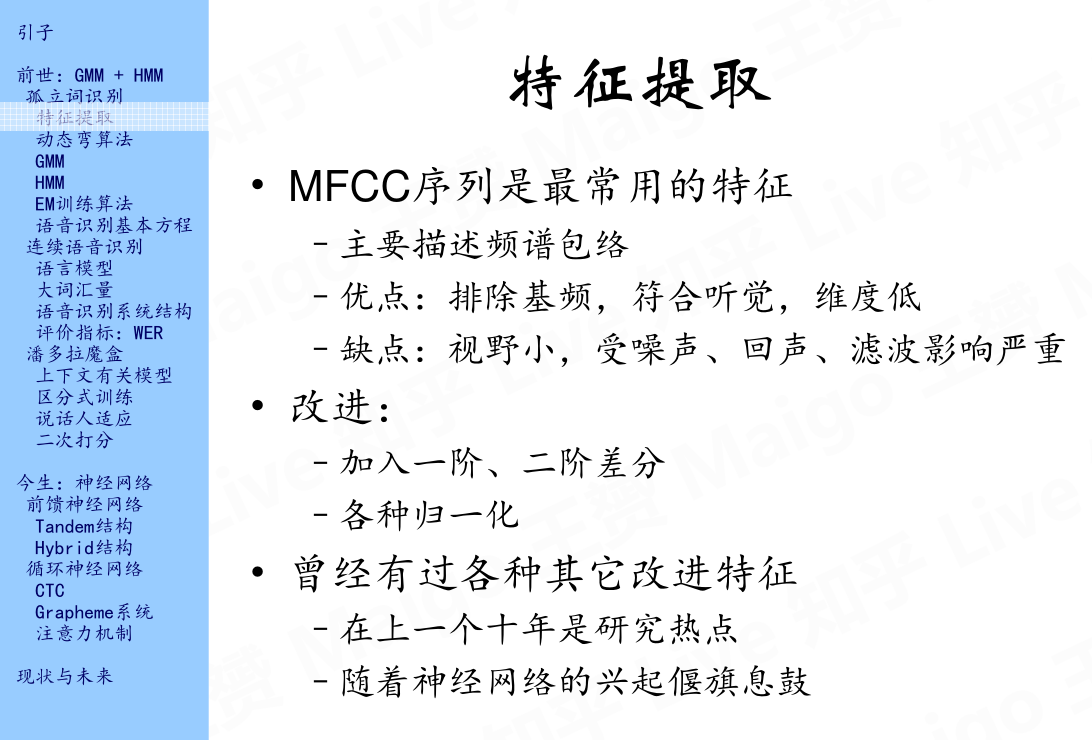## 2.2 动态弯算法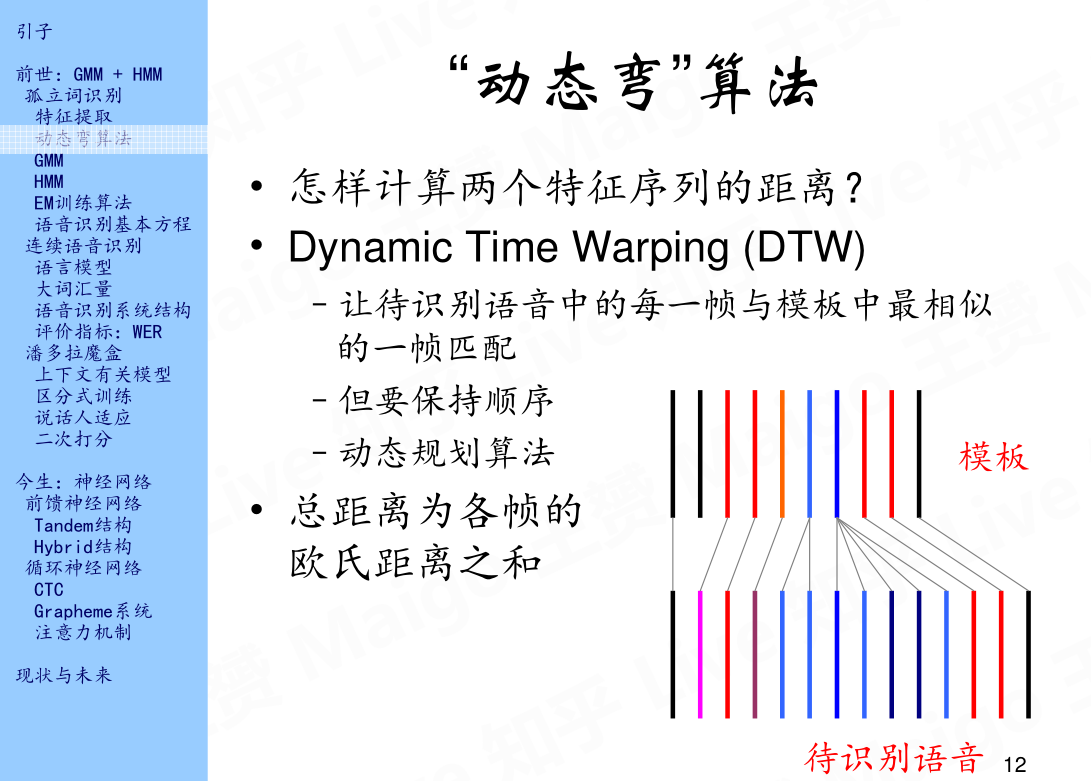## 2.3 GMM(Gaussian mixture model)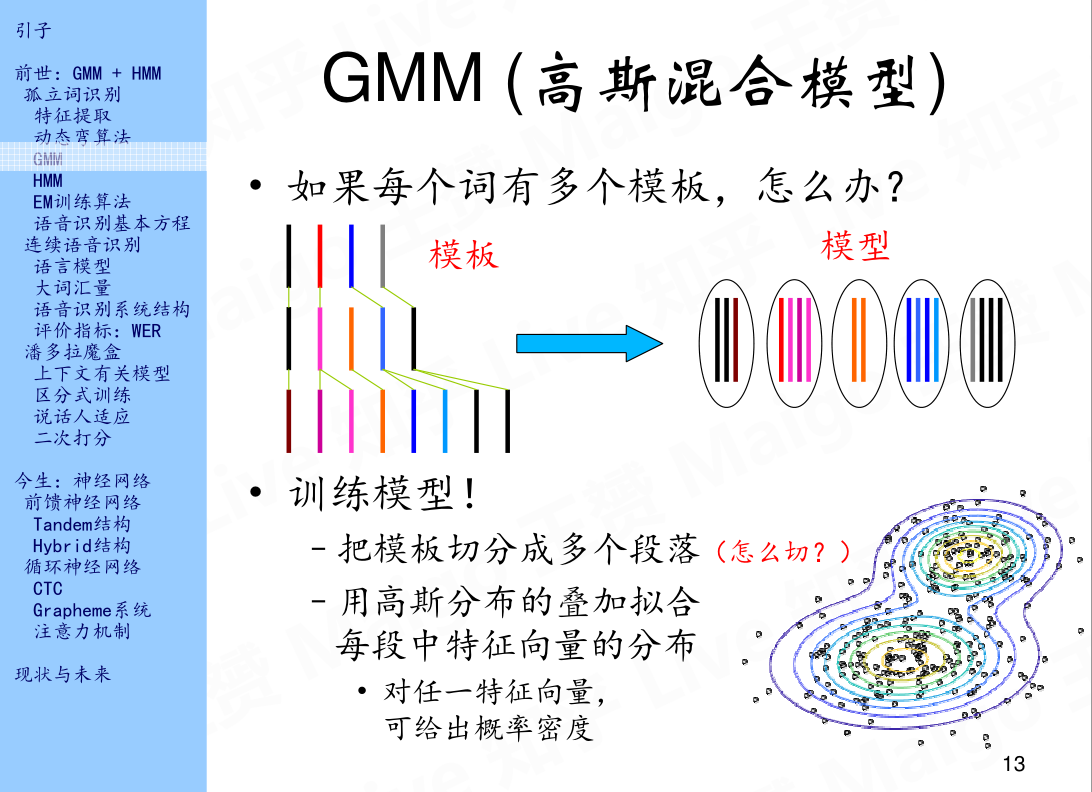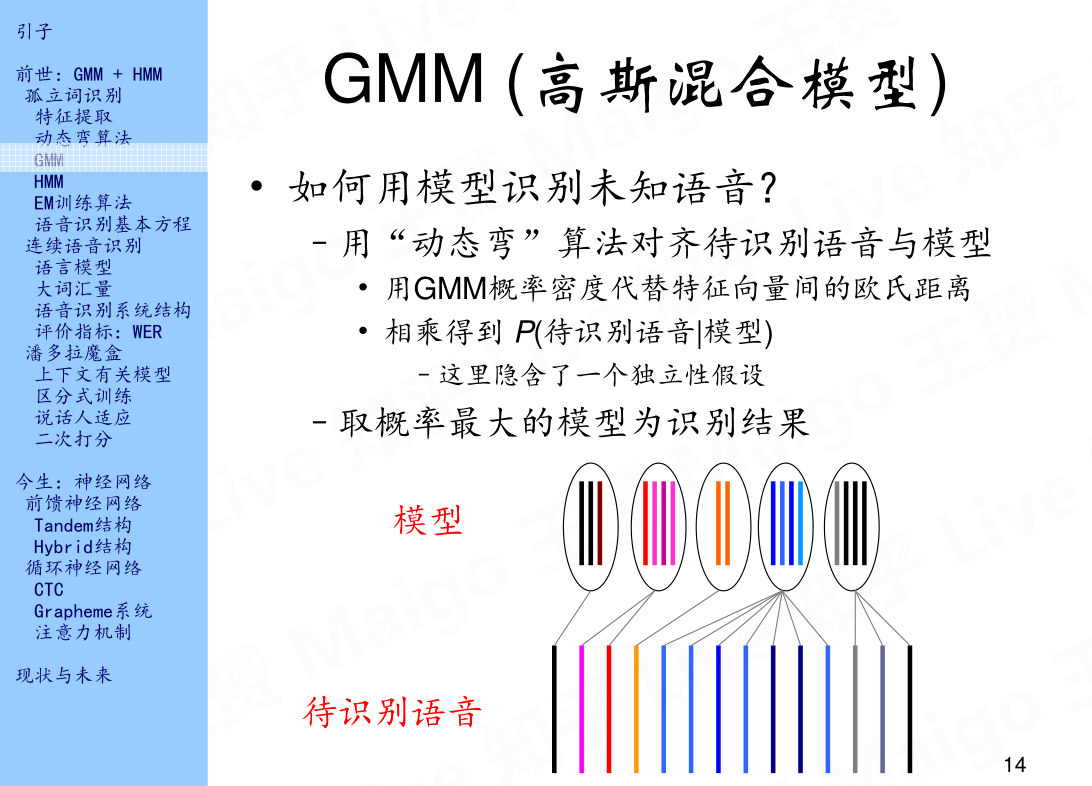## 2.4 HMM(Hidden markov model)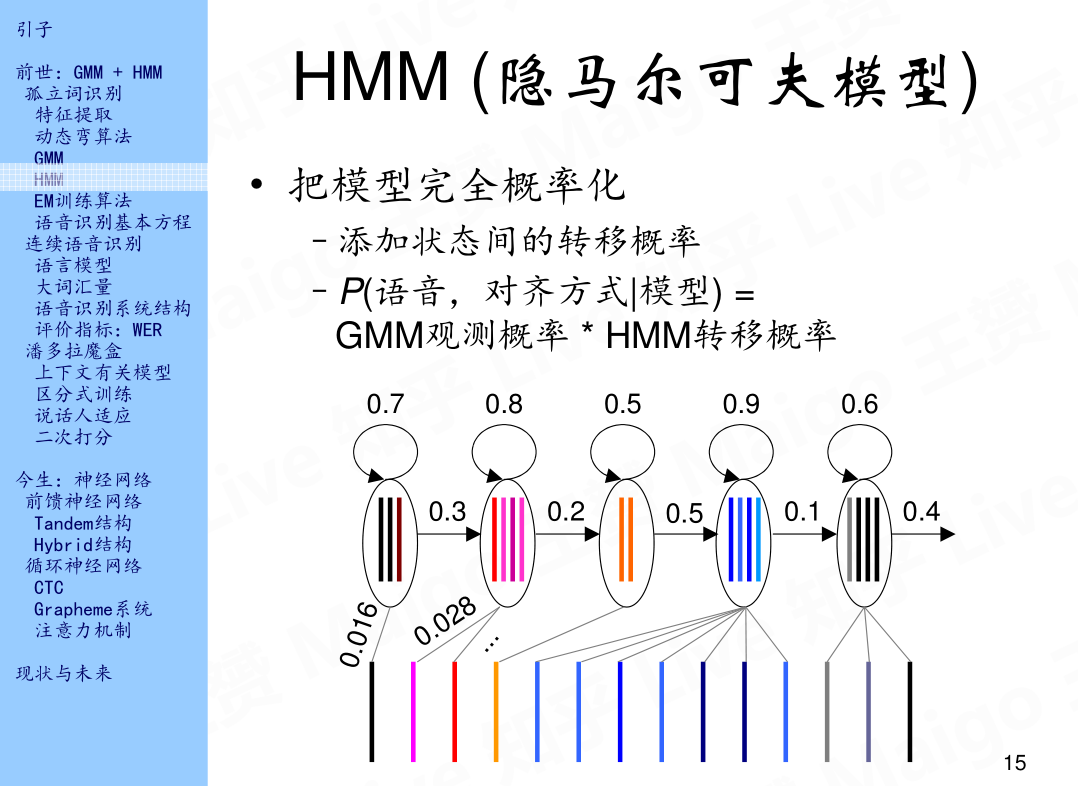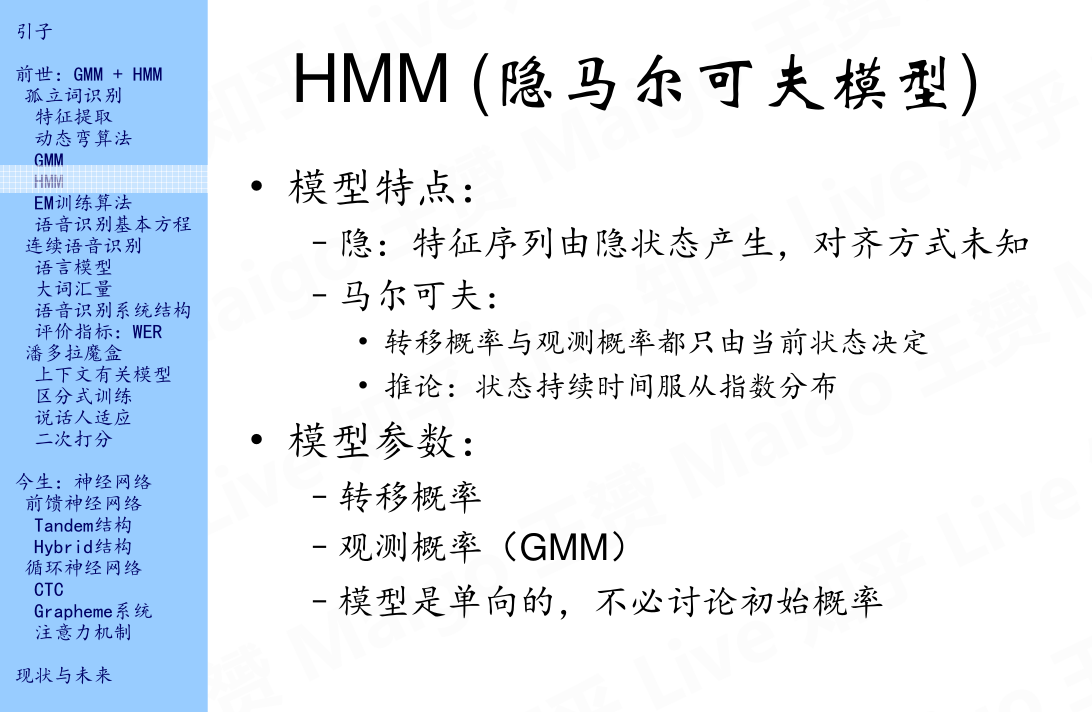HMM主要有两个特点，第一个就是特征序列由隐状态产生，隐状态也就是上面说到的五个状态，为什么称之为隐状态呢，是因为每帧特征向量和状态之间的对齐方式是未知的；另一个特点就是马尔可夫性，这里就不多说了。有关GMM，HMM，包括下面将要说的EM的相关知识，我推荐大家可以看一下悉尼科技大学的徐亦达老师在优酷的一个自频道，里面详细的讲解了概率统计的一些知识，并且每一个概念都有详细的公式推导，值得一看，教学视频见参考文献3。说完模型的特点，接下来说一下模型的参数，实际上HMM是有三个参数的：转移概率，观测概率以及初始概率。但是上面示例中的HMM是单向的，所以初始状态默认为第一个状态，所以，模型的参数就变成了两个：转移概率和观测概率。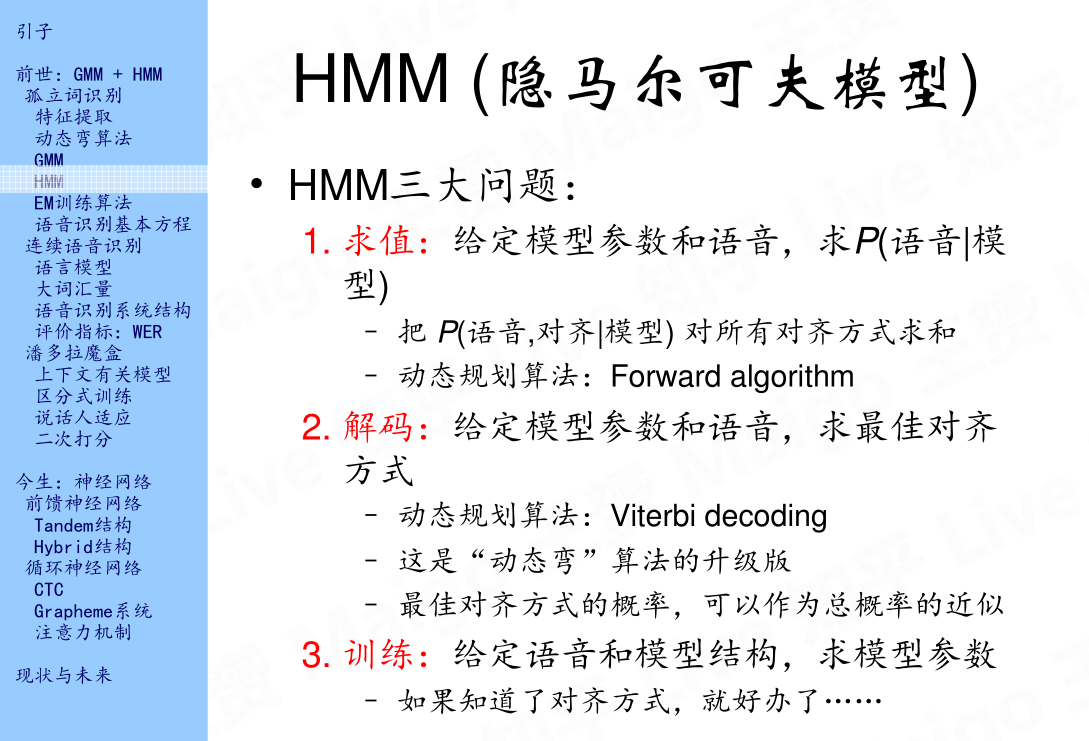## 2.5 EM训练算法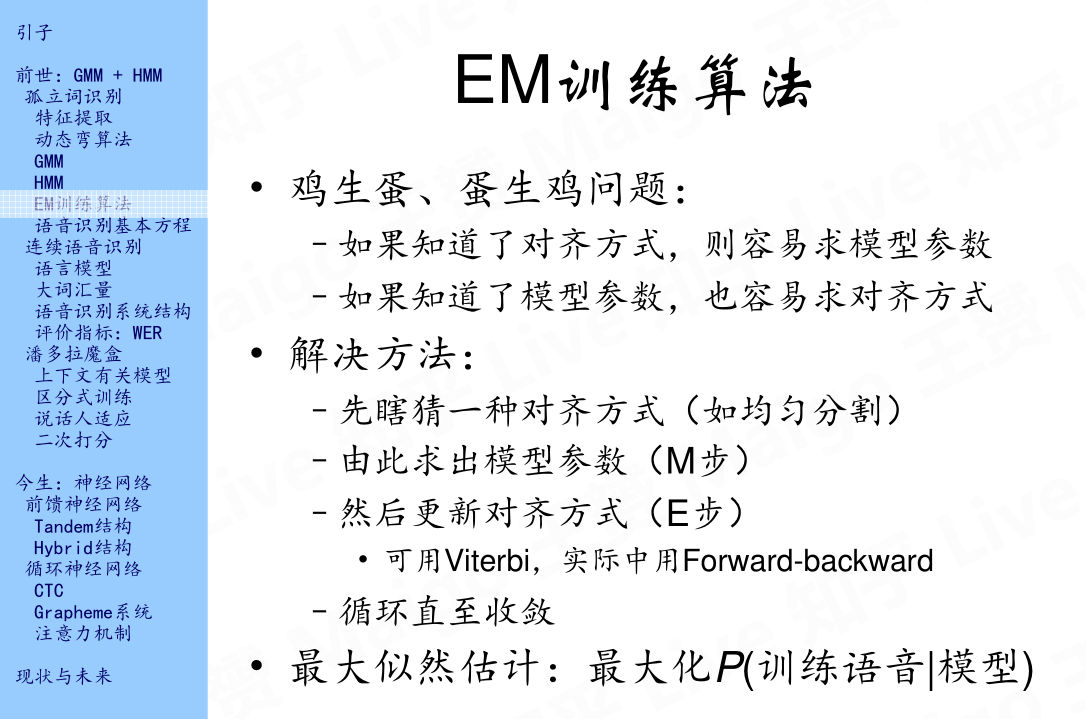## 2.6 语音识别基本方程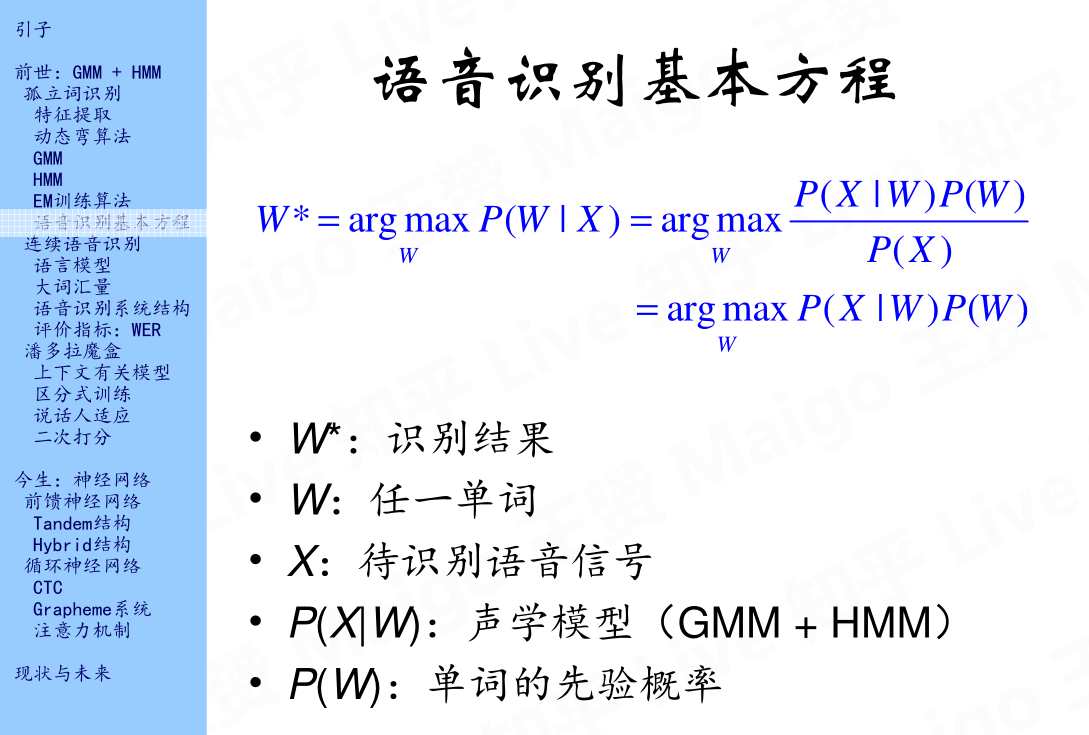# 3.连续语音识别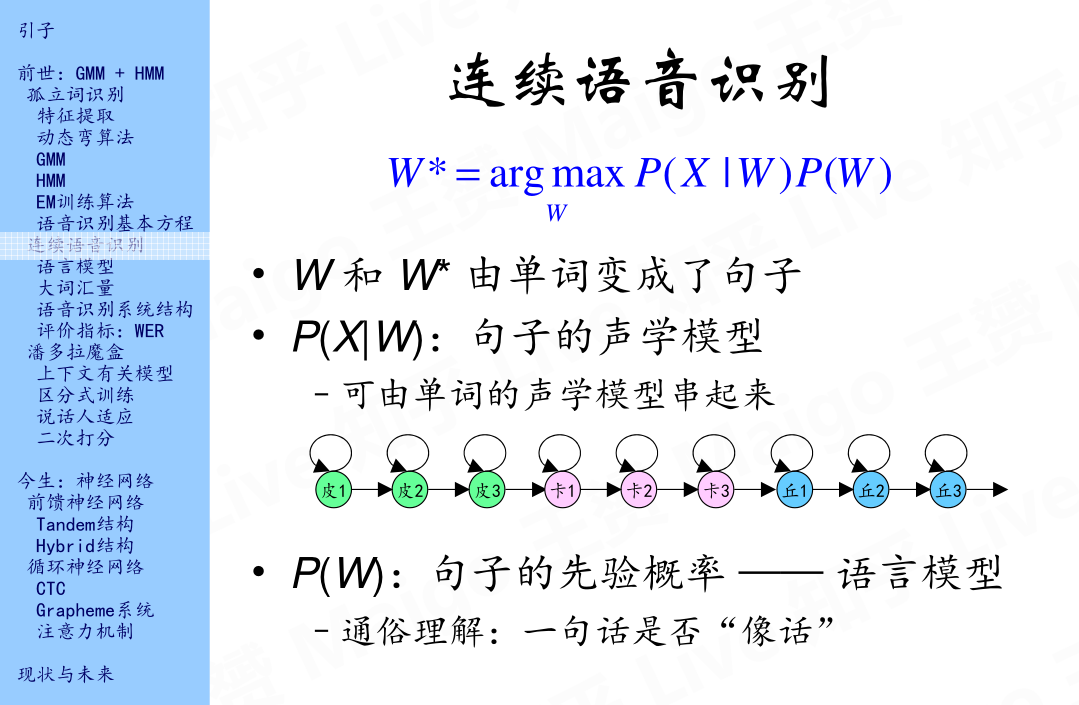## 3.1 语言模型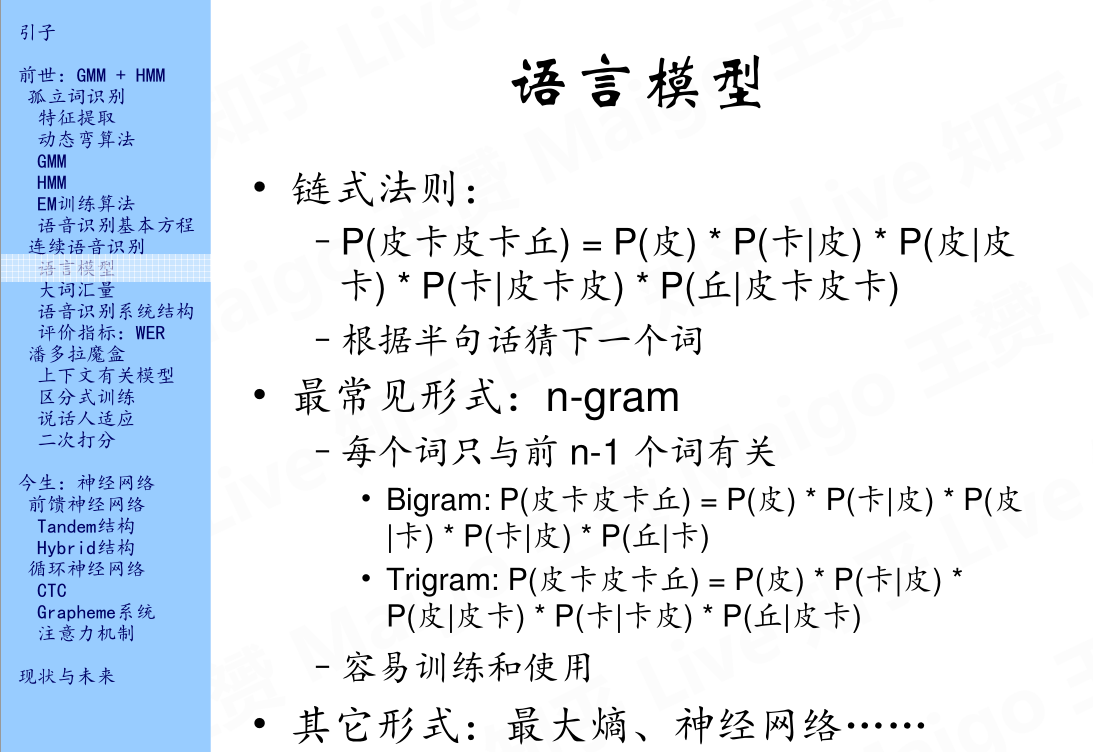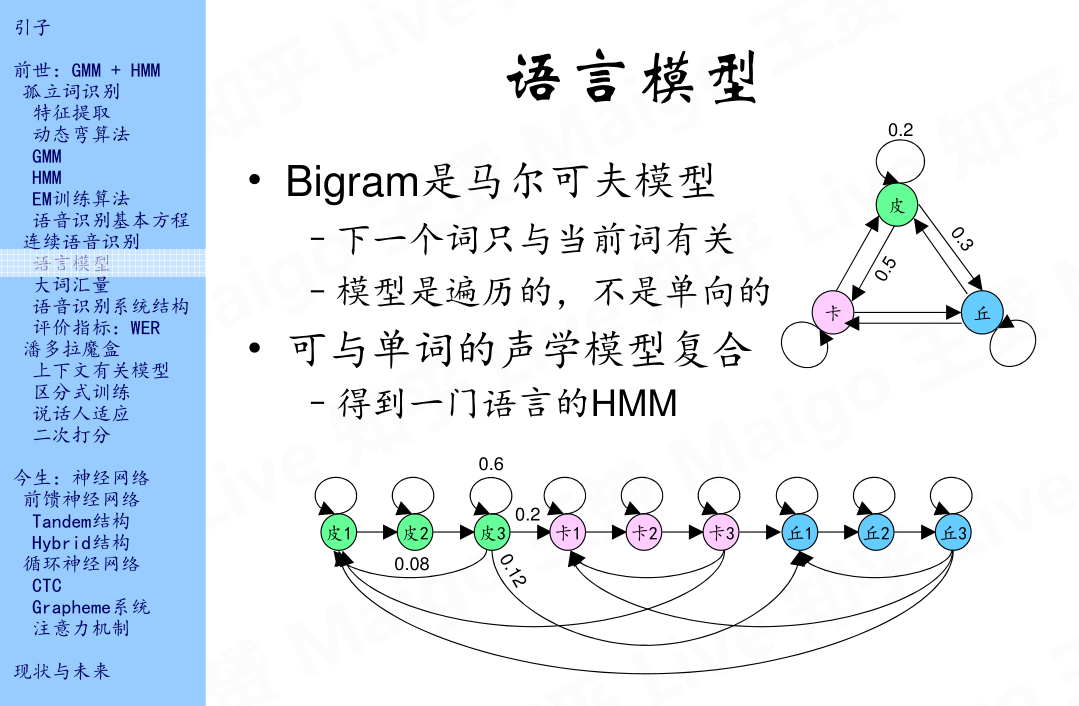## 3.2 大词汇量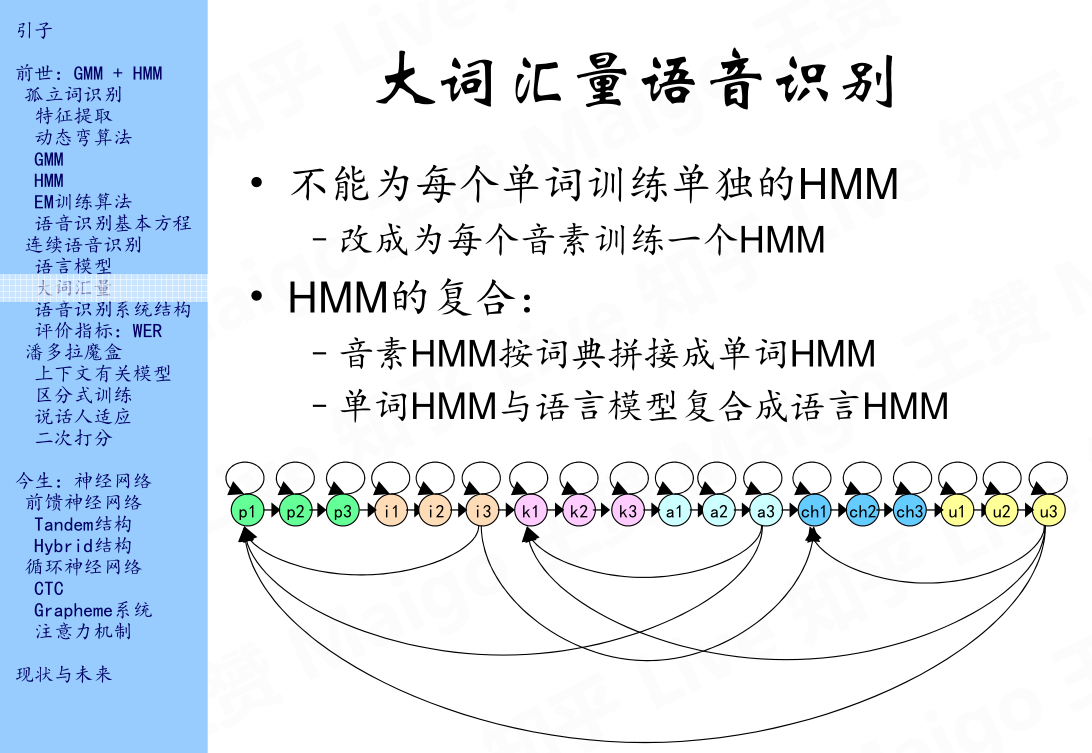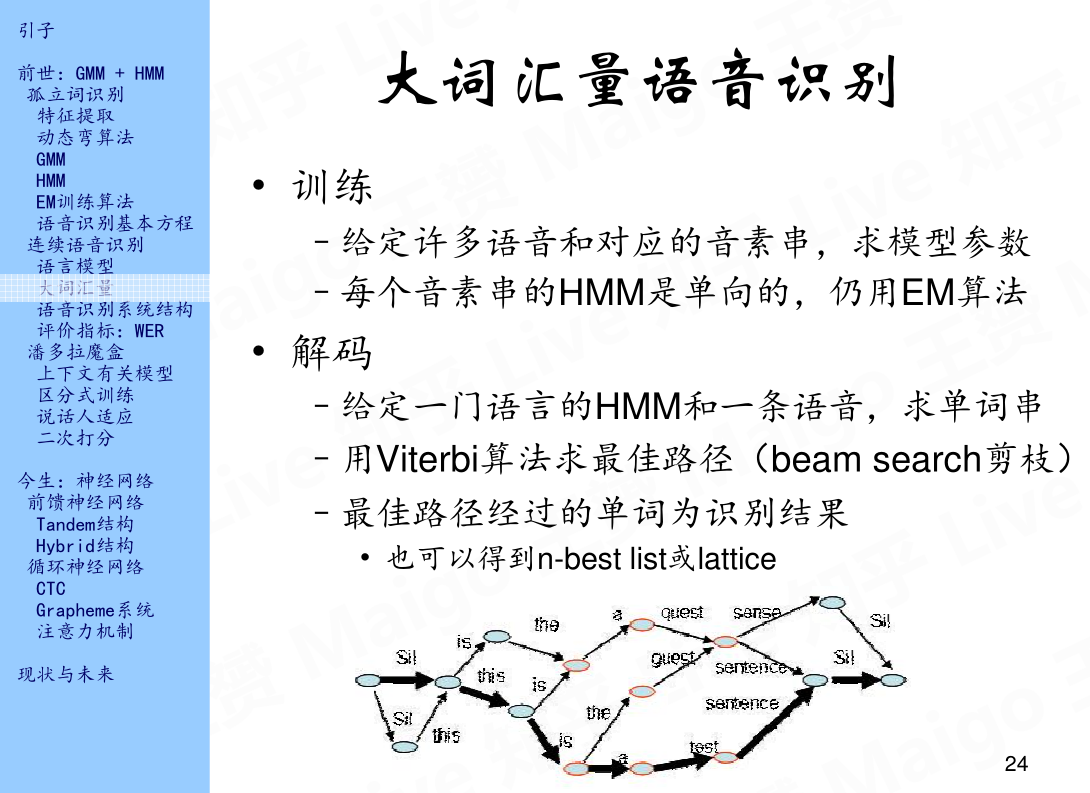## 3.3 语音识别系统结构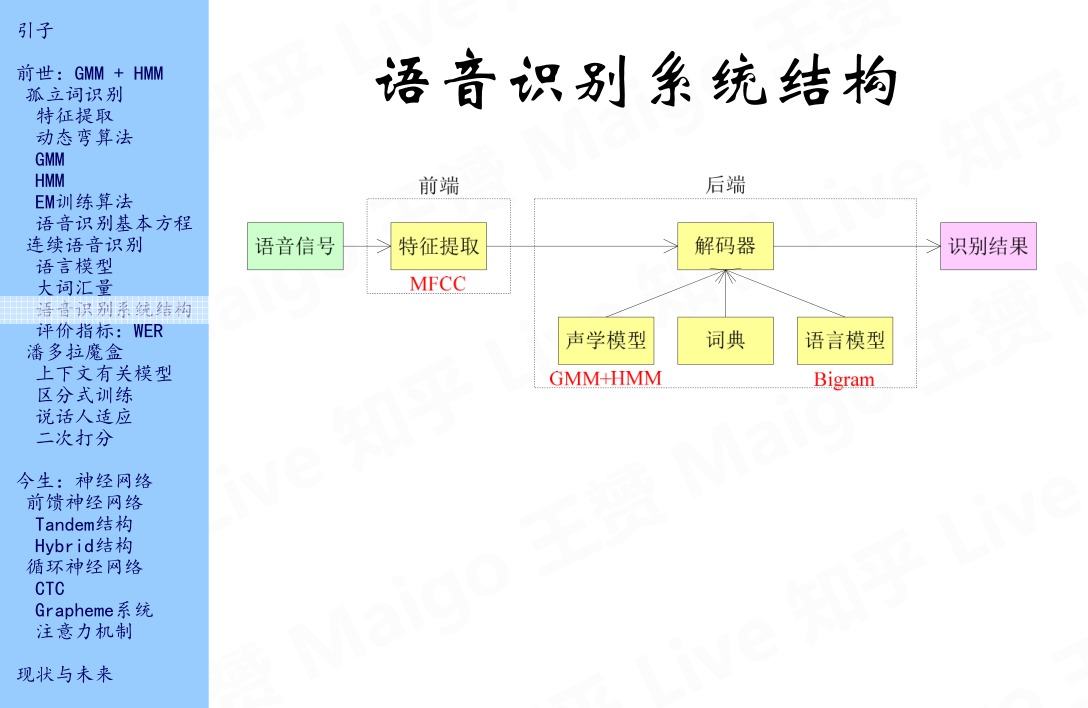## 3.4 评价指标：WER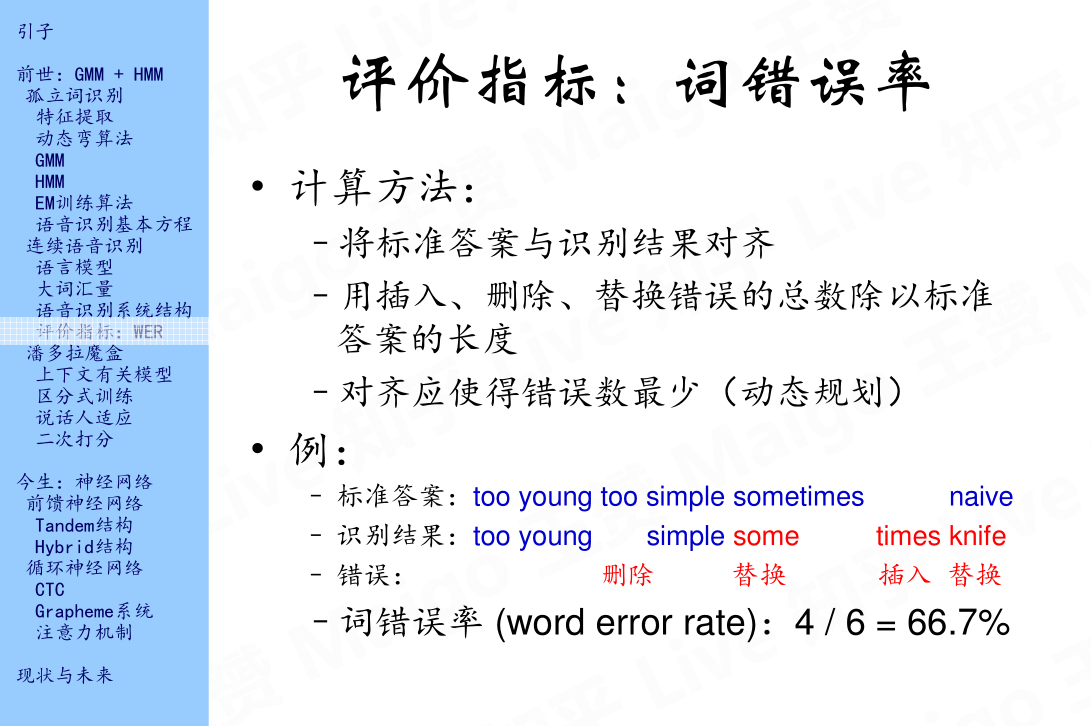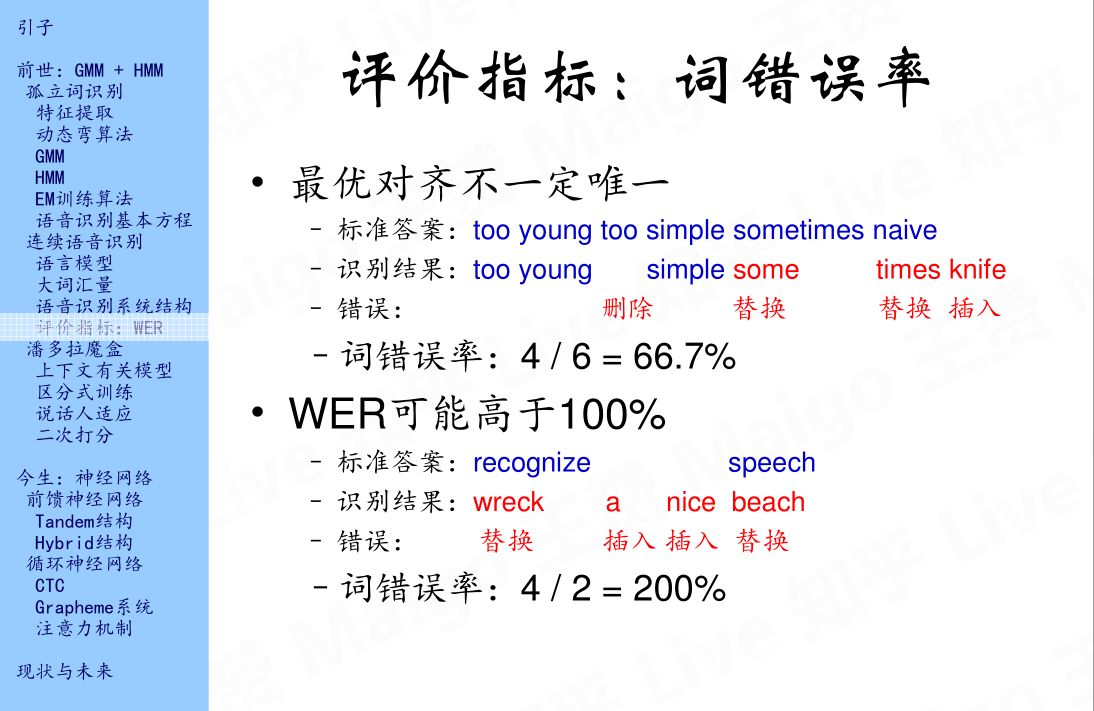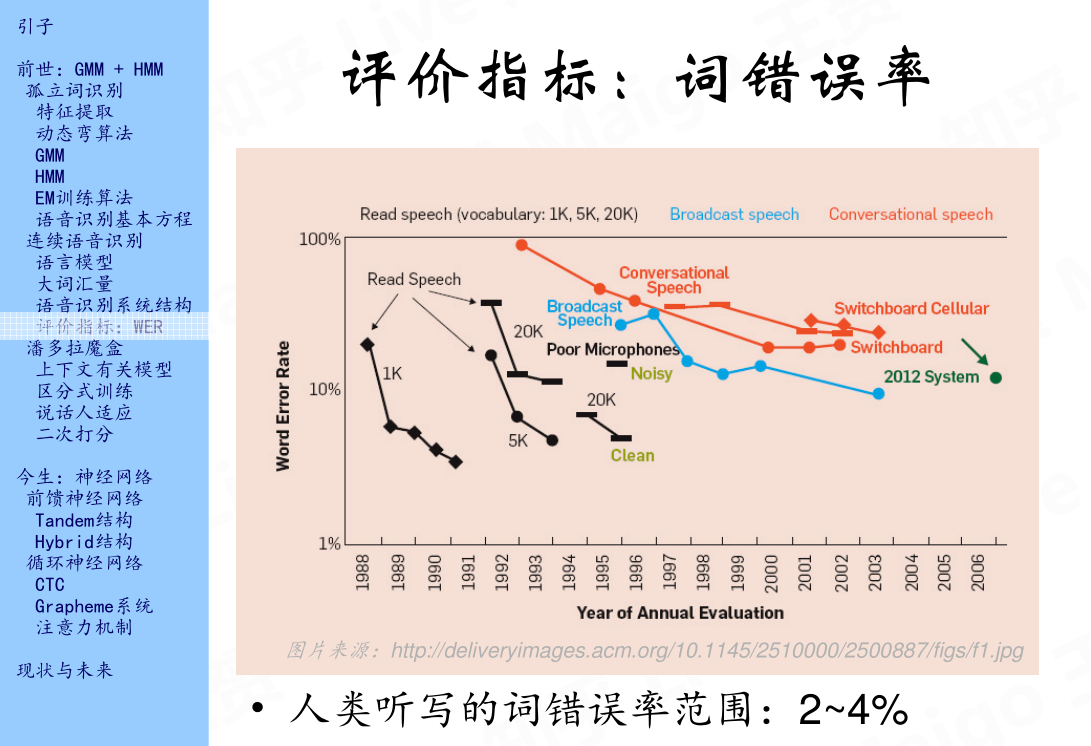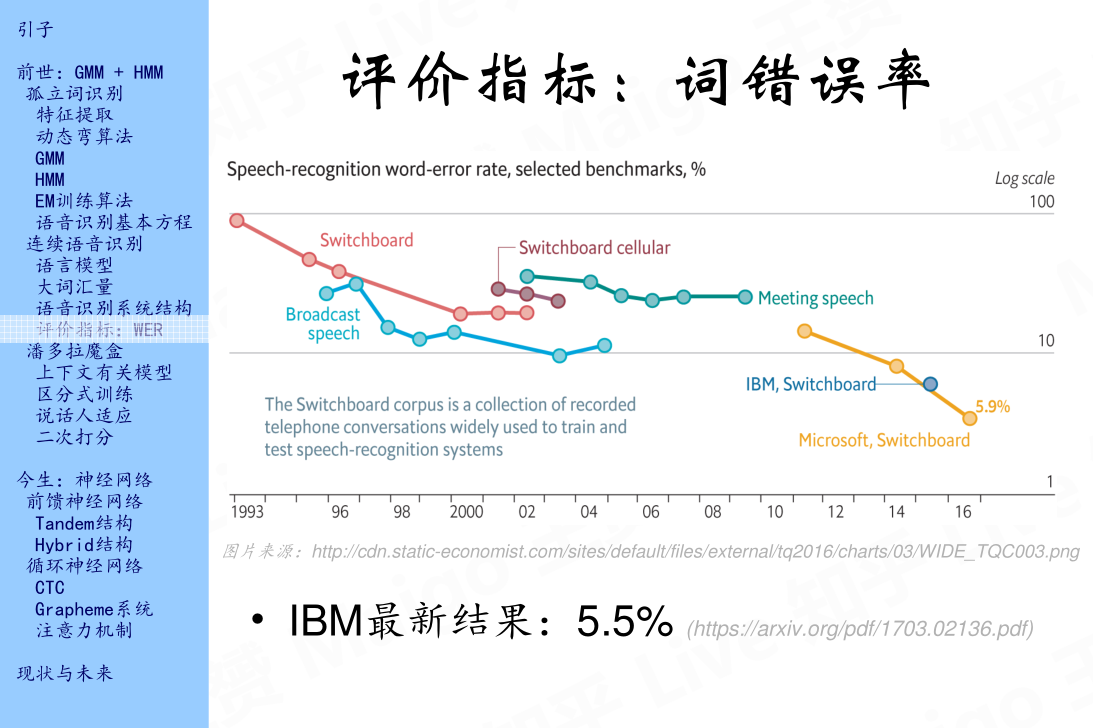# 4. 潘多拉魔盒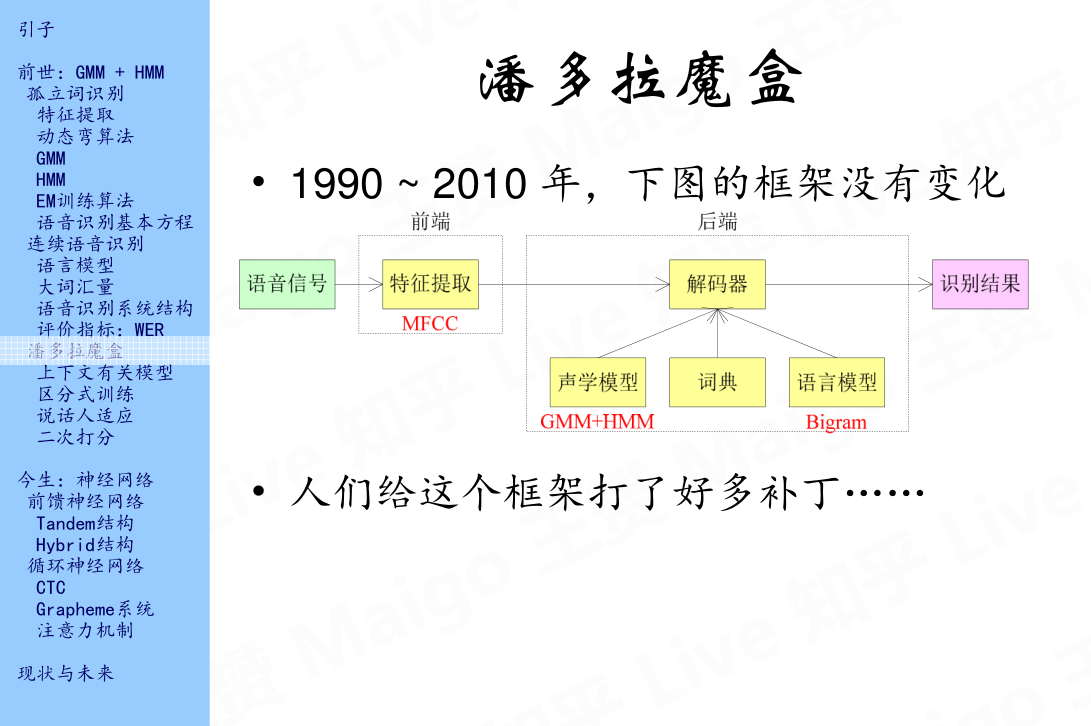## 4.1 上下文有关模型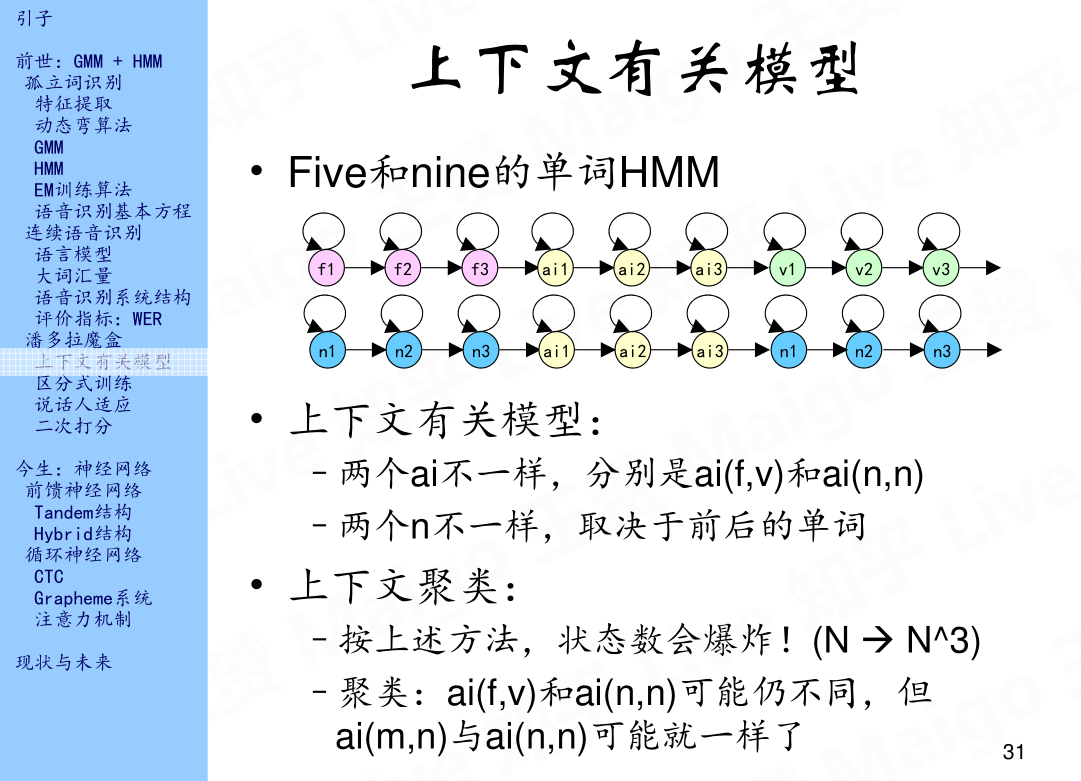## 4.2 区分式训练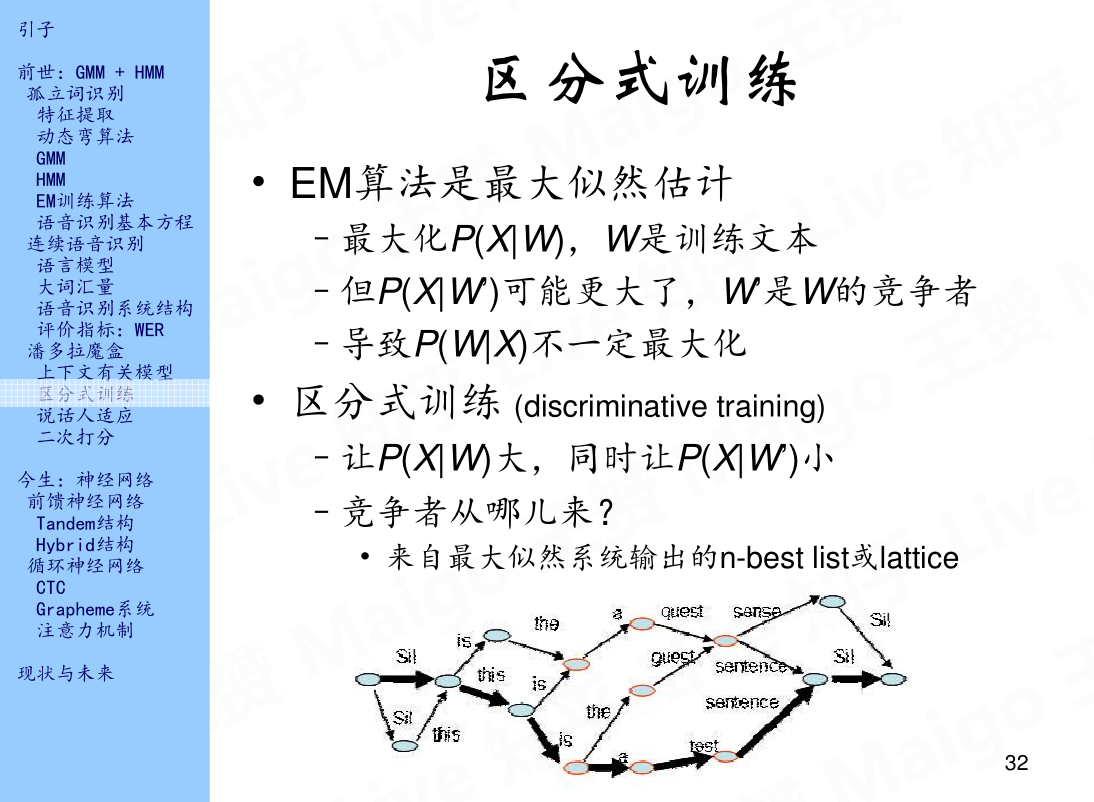## 4.3 说话人适应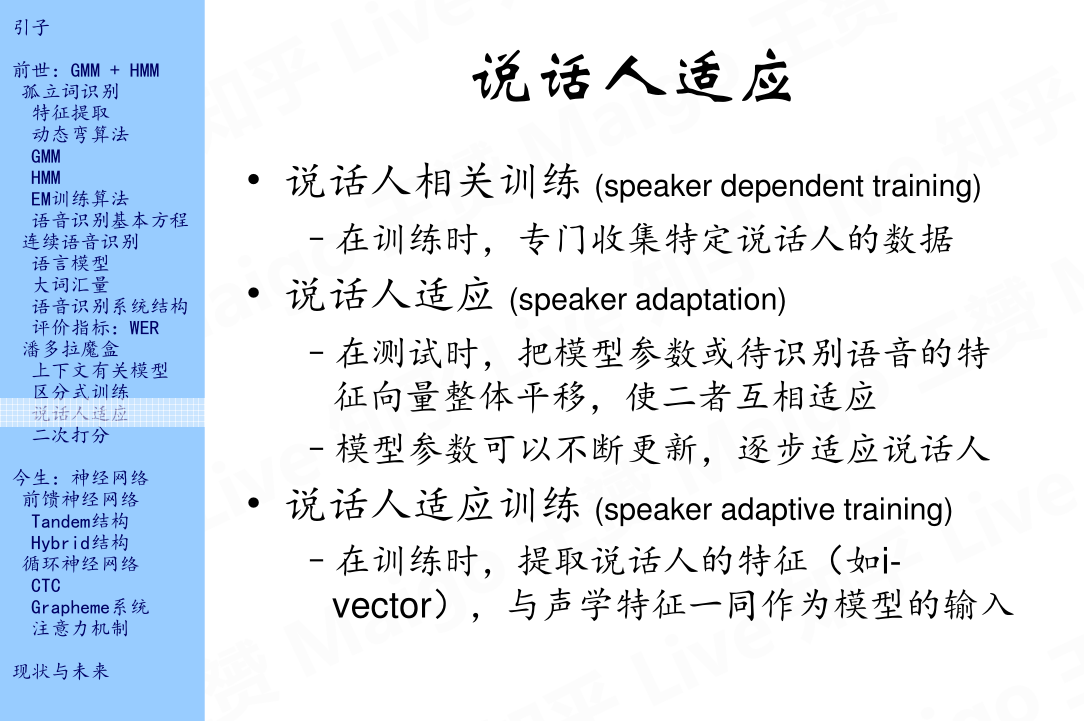## 4.4 二次打分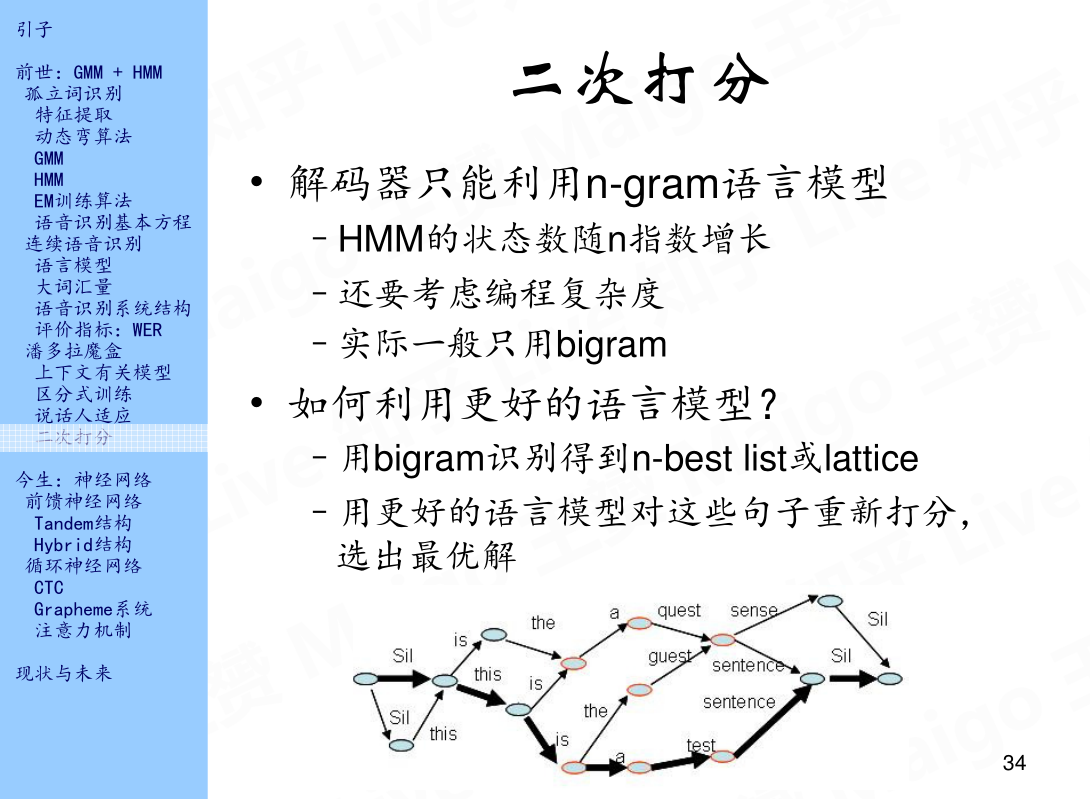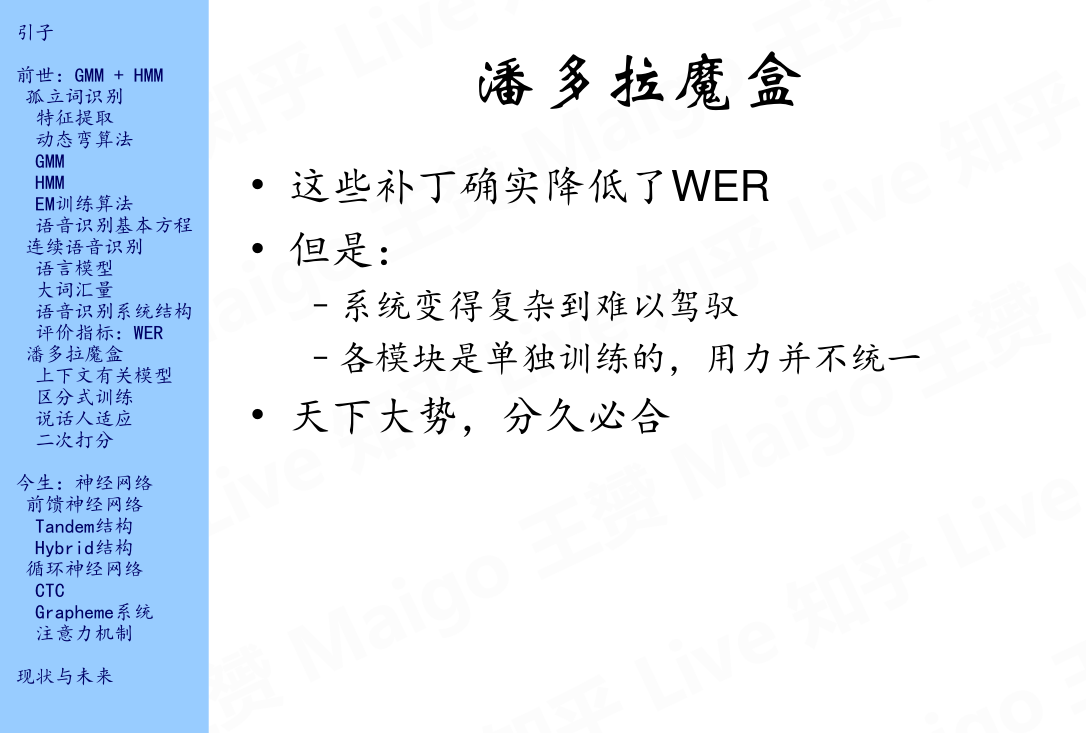# 参考文献

1、语音识别技术的前世今生：https://www.bilibili.com/video/av19158521/

2、傅里叶变换：https://zhuanlan.zhihu.com/p/19763358

3、徐亦达自频道：http://i.youku.com/i/UMzIzNDgxNTg5Ng==?spm=a2hzp.8253869.0.0

2018-10-17 17:28:52 SrdLaplace 阅读数 625
• ###### 云从科技：详解CNN-pFSMN模型以及在语音识别中的应用

近日，云从科技在语音识别技术上取得重大突破，在全球最大的开源语音识别数据集Librispeech上刷新了世界纪录，错词率（Worderrorrate，WER）降到了2.97%，将Librispeech的WER指标提升了25%，大幅刷新原先记录。本视频课程讲解了：大规模词汇连续语音识别，LVCSR前沿与最新进展，和CNN-pFSMN声学模型。

1122 人正在学习 去看看 CSDN讲师

CTC是一种端到端的语音识别技术，他避免了需要字或者音素级别的标注，只需要句子级别的标注就可以进行训练，感觉非常巧妙，也很符合神经网络浪潮人们的习惯。特别是LSTM+CTC相较于之前的DNN+HMM，LSTM能够更好的捕捉输入中的重要的点（LSTM随着状态数目增加参数呈线性增加，而HMM会平方增加），CTC打破了隐马尔科夫的假设，把整个模型从静态分类变成了序列分类。

## 语音识别模型

$p(l|x)=\sum_{\pi=\in B^{-1}(l)}p(\pi|x)$

## 前向后向算法（Forward-Backward Algorithm）

$\alpha(t,s)$可以递推的用$\alpha(t-1,s)，\alpha(t-1,s-1)$计算。

$\alpha(1,1)=y_b^1$

$\alpha(1,2)=y_{l_1}^1$

$\alpha(1,u)=0,u>2$

$\alpha(t,0)=0$

$\alpha(t,u)=0,u<U'-2(T-t)-1$

$U'$表示序列$2|l_{1:u}|+1$

$\alpha(t,u)=y_{l'_u}^t\sum_{i=f(u)}^u\alpha(t-1,i)$

$f(u)=\{\begin{array}{} u-1 & if~l'_u=blank~or~l'_{u-2}=l'_u\\u-2 & otherwise \end{array}$

$\beta(T,U')=1$

$\beta(T,U'-1)=1$

$\beta(T,u)=0,u<U'-1$

$\beta(t, u)=0,u>2t$

$\beta(t,u)=\sum_{i=u}^{g(u)}\beta(t+1,i)y^{t'+1}_{l^u_t}$

$g(u)=\{\begin{array}{} u+1 & if~l'_u=blank~or~l'_{u+2}=l'_u\\u+2 & otherwise \end{array}$

$ln(a+b)=ln~a+ln(1+e^{ln~b-ln~a})$

## Loss Function

the CTC loss函数$L(S)$被定义为训练集$S$种正确标签的negative log probability：

$L(S)=-ln\prod_{(x,z)\in S}=-\sum_{(x,z)\in S}ln~p(z|x)$

$L(x,z)=-ln~p(z|x)$

$L(S)=\sum_{(x,z)\in S}L(x,z)$

$\frac{\partial L(S)}{\partial w}=\sum_{(x,z)\in S}\frac{\partial L(x,z)}{\partial w}$

$\alpha(t,u)\beta(t,u)=\sum_{\pi_t=z_u,B(\pi)=z}\prod_{t=1}^Ty_{\pi_t}^t=\sum_{\pi_t=z_u,B(\pi)=z}p(\pi|x)$

$p(z|x)=\sum_{u=1}^{|z'|}\alpha(t,u)\beta(t,u)$

$L(x,z)=-ln~\sum_{u=1}^{|z'|}\alpha(t,u)\beta(t,u)$

$\frac{\partial L(x,z)}{\partial y_k^t}=-\frac{\partial ln~p(z|x)}{\partial y_k^t}=-\frac{1}{p(z|x)}\frac{\partial p(z|x)}{\partial y_k^t}$

$\frac{\partial p(z|x)}{\partial y_k^t}=\frac{1}{y_k^t}\sum_{u\in B(z,k)}\alpha(t,u)\beta(t,u)$

$\frac{\partial L(x,z)}{\partial y_k^t}=-\frac{1}{p(z|x)\cdot y_k^t}\sum_{u\in B(z,k)}\alpha(t,u)\beta(t,u)$

$\frac{\partial L(x,z)}{\partial a_k^t}=-\sum_{k'}\frac{\partial L(x,z)}{\partial y_{k'}^t}\frac{\partial y_{k'}^t}{\partial a_k^t}$

$y_k^t=\frac{e^{a_k^t}}{\sum_{k'}e^{a_{k'}^t}}$

$\frac{\partial y_{k'}^t}{\partial a_k^t}=y_{k'}^t\delta_{kk'}-y_{k'}^ty_{k}^t$

$\frac{\partial L(x,z)}{\partial a_k^t}=y_k^t-\frac{1}{p(z|x)}\sum_{u\in B(z,k)}\alpha(t,u)\beta(t,u)$

## decoder

$h(x)=argmax_{l}p(l|x)$

### 最佳路径解码（best path decoding）

$h(x)=B(\pi^*),s.t.,\pi^*=argmax_{\pi}p(\pi|x)$

### 前缀查找解码（prefix search decoding）

$l^*$表示当前找到最好的的序列，$p^*$表示以$p^*$为开头的序列可能性最高，当$p^*$为开头的序列可能性小于表示当前找到最好的的序列$l^*$出现的可能性时停止循环，认为$l^*$就是最有可能的序列。循环过程中，对于最有可能的前缀尝试后面加入每一个可能的字符，然后计算加入这个字符之后新的字符串出现的概率和以新的字符串为前缀的概率，当新的字符串出现的概率大于$l^*$时更新$l^*$，把以新的字符串为前缀的概率大于$l^*$出现的概率的前缀加入带备选集合，循如果发现剩下字符搭配$p^*$做前缀的概率之和小于$l^*$出现的概率，停止循环，节约计算。循环完毕后把旧的$p^*$从备选集合中剔除，再把集合中概率最大的前缀作为$p^*$
$\gamma(p_n,t)$表示序列$p$$t$个输出非blank，$\gamma(p_b,t)$表示序列$p$$t$个输出为blank。

## tensorflow中的CTC

tf.nn.ctc_loss(labels, inputs, sequence_length)


labels: 标签，一个稀疏矩阵
inputs：模型输出的logits

decoded, _ = tf.nn.ctc_beam_search_decoder(logits, sequence_len, merge_repeated=False)
decoded, _ = tf.nn.ctc_greedy_decoder(logit, sequence_len, merge_repeated=False)


logits：模型输出的logits
sequence_len：实际的长度（tensorflow静态图模型，告诉他实际的音频长度多长）
merge_repeated：把连续输出相同的合并，不同于ctc_merge_repeated，ctc_merge_repeated是把中间没有blank的合并。

## 音识别模型小型综述

• 2011-2012年：发现把GMM换成DNN有30%的提升
• 2013年：发现调整激活函数Maxout对数据量较少的情况有所改善，对大数据量不一定有显著帮助
• 2013-2014年：浅层DNN升级为浅层CNN
• 2014年：开始尝试开始做RNN，尤其是LSTM
• 2014年底2015年初：Hinton 的博士后 Alex Graves，把以前做手写体识别的LSTM 加 CTC 的系统应用在了语音识别领域，在 TIMIT 上做了结果，紧接着谷歌认为这个很有前途，就开始把这些技术推广在大数据上面。
• 2015年：研究者发现 CNN 在时间上做卷积和 LSTM 有很多相似之处但各有特点。LSTM 更擅长做整个时间域的信息整合，因为 CNN 有很强的平移的不变性，那么鲁棒性更强
• 2016年：Deep CNN，WaveNet 等深层 CNN

## 实现的乞丐版语音识别模型

model: 三层lstm模型
loss function: ctc loss
feature: 13阶mfcc系数+2阶动态因子（39）

## 参考文献

Graves A, Fernandez S, Gomez F, et al. Connectionist temporal classification: labelling unsegmented sequence data with recurrent neural networks[C] Proceedings of the 23rd international conference on Machine learning. ACM, 2006: 369-376.

2019-07-27 15:38:04 alice_tl 阅读数 486
• ###### 云从科技：详解CNN-pFSMN模型以及在语音识别中的应用

近日，云从科技在语音识别技术上取得重大突破，在全球最大的开源语音识别数据集Librispeech上刷新了世界纪录，错词率（Worderrorrate，WER）降到了2.97%，将Librispeech的WER指标提升了25%，大幅刷新原先记录。本视频课程讲解了：大规模词汇连续语音识别，LVCSR前沿与最新进展，和CNN-pFSMN声学模型。

1122 人正在学习 去看看 CSDN讲师

### 声波的概念

 声波指标 概念 分类 频率 每秒钟振动的次数 可听声：20-20000HZ 超声：>20000HZ 次声：<20HZ 声压 疏密波压力的大小 与声音的频率和人的年龄有关，一定范围内，声压越大清晰度越大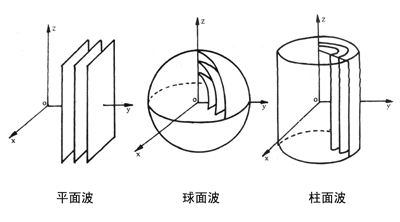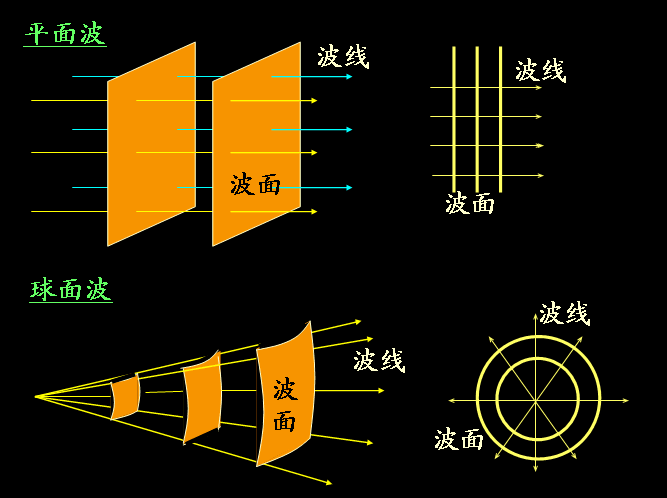### 声音四要素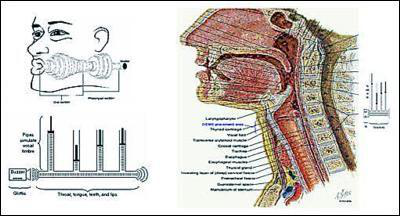要素 概念 效果 举例 音高 表现语音的字调与语调 也叫音调，主要取决于声波基频的高低 是人耳对声音高低的主观感受，频率越高音调越高 人耳听觉范围20～20kHz 音长 对语言节奏的快慢，字与句之间的长短关系等加以准确地计量 主要取决于声波的持续时间 音强 单位时间内通过声音的能量。也叫响度和音量 主要取决于声波振幅的大小，显示语音的重音、轻音等强弱变化 正常人的范围在0dB-140dB（分贝） 音色 也叫音频，取决于不同的泛音 不同的声音频率在波形上表现出不同的特性 辅音的发音，发音的部位，元音的音色，语调的高低，轻重音的强度，高中低音色，男女音色不同，不同乐器的音色。由发声物体本身材料、结构决定。

### 音高和频率

 分类 标准 举例 特低频（ULF） 3~30千赫（kHz） 闷雷声、风声，人声在这个频段没有声音 低频（LF） 30~300千赫（kHz） 钢琴低音、低音提琴，男低音可达到某个频率的某些部分 中频（MF） 300~3000千赫（kHz） 电话、收音机，人耳最容易接受的声音频段 甚高频（VHF） 30~300兆赫（MHz） 特高频（UHF） 300~3000兆赫（MHz） 超高频（SHF） 3~30秭赫（GHz） 高频噪音 极高频（EHF） 30~300秭赫（GHz）

### 环境和分贝

 分贝（db） 环境 分贝（db） 环境 110 列车通过铁路桥时,正下方 60 安静的街头 100 地铁行车时,车厢内 50 安静的办公室 90 公共汽车内 40 安静的住宅小区，白天 80 白天十字路口 30 安静的住宅小区，夜晚 70 普通讲话 20 飞机起飞着路时，正下方

### 声学的基本术语

phone，根据语音的自然属性划分出来的最小语音单位，依据音节里的发音动作来分析，一个动作构成一个音素。相同发音动作发出的音就是同一音素，不同发音动作发出的音就是不同音素。

• 元音音素：发音时气流在口腔、咽头不受阻碍而形成的音叫元音。
• 辅音音素：发音时气流在口腔、咽头受到一定程度的阻碍而形成的音叫辅音。

28个辅音音素，其中十个清辅音与十个浊辅音恰好成对，以及8个音标。

 英语音素分类 分类明细 数量 分类明细 具体音素 元音音素 单元音 12 前元音 /iː/，/ɪ/，/e/，/æ/ 中元音 /ɜː/，/ə/ 后元音 /ɑː/，/ʌ/，/ɔː/，/ɒ/，/uː/，/ʊ/ 双元音 8 合口双元音 /aɪ/，/eɪ/，/aʊ/，/əʊ/，，/ɔɪ/ 集中双元音 /ɪə/，/eə/，/ʊə/ 辅音音素 清辅音 10 /p/，/t/，/k/，/f/，/s/，/θ/，/ʃ/，/tʃ/，/ts/，/tr/ 浊辅音 10 /b/，/d/，/g/，/v/，/z/，/ð/，/ʒ/，/dʒ/，/dz/，/dr/ 音标 8 /m/，/n/，/l/，/ŋ/，/h/，/r/，/j/，/w/

ma和mi中的m就是同一个因素。

Putonghua（普通话）中包含p、u、t、o、ng、h、u、a共8个因素。

Senone，有时候，音素会被放在上下文中考虑，这样就形成了三元音素或者多元音素。但它与亚音素不同，他们在波形中匹配时长度还是和单一音素一样。

syllables，由音素phones构成的亚单词单元，称为音节。通俗的讲，指由一个或数个音素组成的语音结构基本单位。

Sound Channel，是指声音在录制或播放时在不同空间位置采集或回放的相互独立的音频信号，所以声道数也就是声音录制时的音源数量或回放时相应的扬声器数量。

### 谐波

harmonicwavelength，是一个数学或物理学概念，是指周期函数或周期性的波形中能用常数、与原函数的最小正周期相同的正弦函数和余弦函数的线性组合表达的部分。